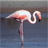# New to Qlik Sense

If you’re new to Qlik Sense, start with this Discussion Board and get up-to-speed quickly.

Announcements
Read about the latest Qlik Community enhancements on the Community News blog!
cancel
Showing results for
Did you mean:Contributor II

## Rank in a KPI chart

Hi,

Is it possible to show a tied rank in a KPI chart?

Below is the same measure but one is in a table and one is in a KPI. I can't get the KPI to display the 8-14.

I have already tried to change the format in the script e.g. rank(xxx ,0,0) but I only get either 8 or 14 - depending on the formatting).

ThanksLabels (2)

• ### Ranks

1 Solution

Accepted SolutionsMVP

Can you try this

``````MinString({\$<Response_% = p([Company name])>} Aggr(
Rank(Sum({<Response_%, QuestionID_% = {'Q1'} ,[Cle2_%] = {'111'}>} [Weighting_factor_%]))
, [Response_%]))``````

6 RepliesMVP

What is the expression used in the chart and in the kpi object?Contributor II
Author

My expression is:

Only({\$<Response_% = {"\$(=GetFieldSelections([Company name]))"}>}

aggr({<Response_%>}rank(

SUM({<Response_%>}{<QuestionID_% = {'Q1'} ,[Cle2_%] = {'111'} >} [Weighting_factor_%])
/
SUM(TOTAL {<Response_%>} {<QuestionID_% = {'Q1'} ,[Cle2_%] = {'111'} >}Weighting_factor_%) ), [Response_%]))

ThanksMVP

Can you try this

``````MinString({\$<Response_% = p([Company name])>} Aggr(
Rank(Sum({<Response_%, QuestionID_% = {'Q1'} ,[Cle2_%] = {'111'}>} [Weighting_factor_%]))
, [Response_%]))``````Contributor II
Author

Thanks!

I still just get the lowest value in the KPI chart, but the range in the table:MVP

Change Number Formatting to Measure expressionContributor II
Author

Fab! thanks very muchTags
Community Browser